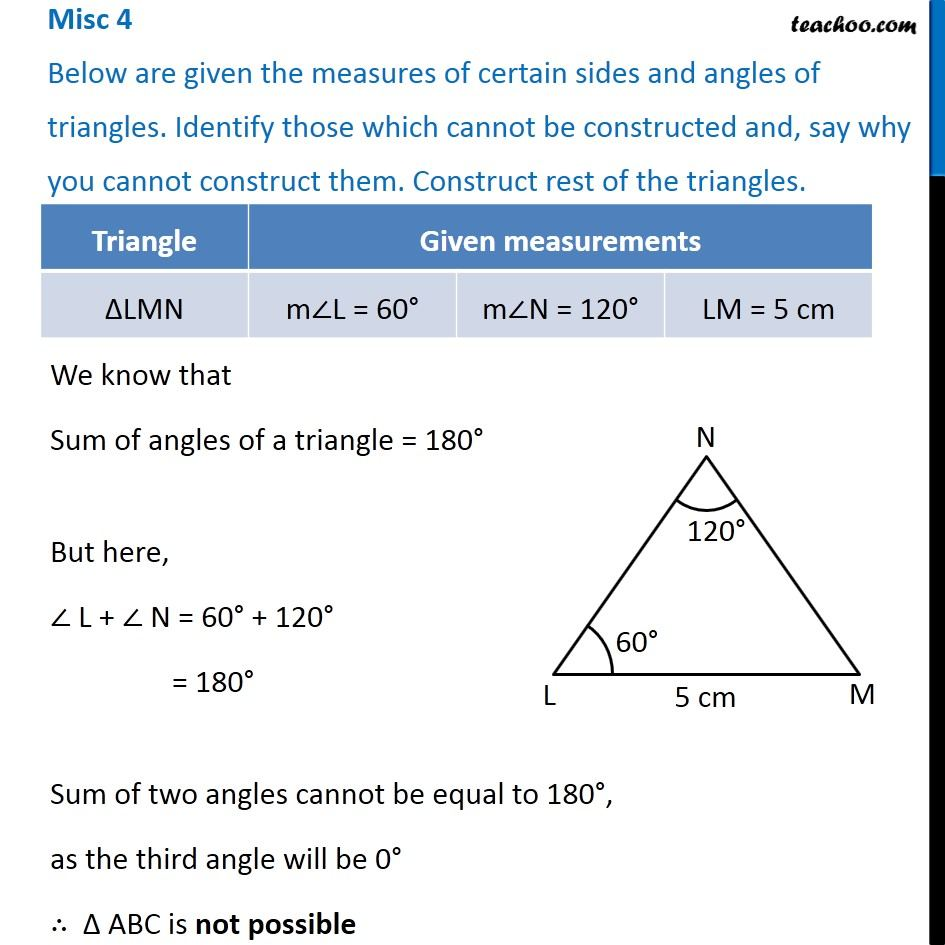Miscellaneous

Practical Geometry
Serial order wiseLearn in your speed, with individual attention - Teachoo Maths 1-on-1 Class

### Transcript

Misc 4 Below are given the measures of certain sides and angles of triangles. Identify those which cannot be constructed and, say why you cannot construct them. Construct rest of the triangles. We know that Sum of angles of a triangle = 180° But here, ∠ L + ∠ N = 60° + 120° = 180° Sum of two angles cannot be equal to 180°, as the third angle will be 0° ∴ Δ ABC is not possible